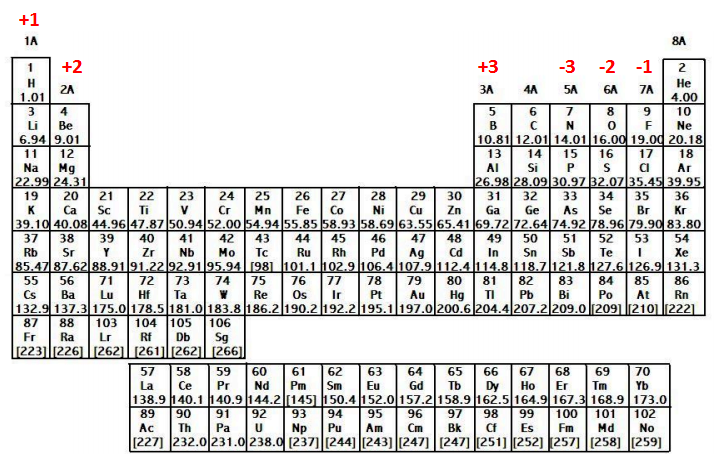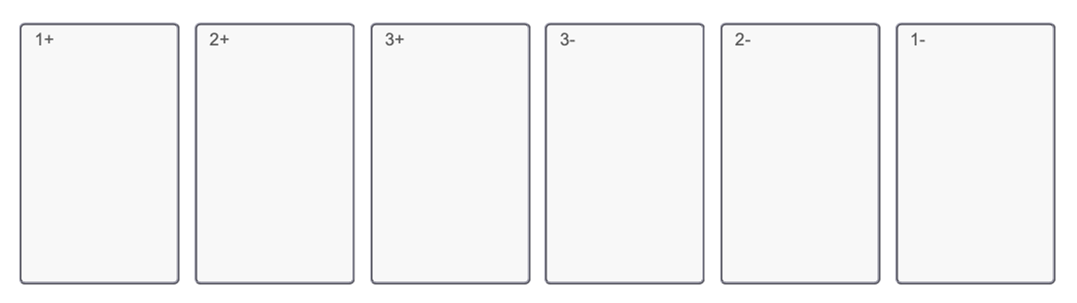# Problem: Part B. What is the charge on the most stable ion of each of the following elements?Ba, N, O I, K, Al, Mg, SDrag each item to the appropriate bin. An atom is made up of a positively-charged nucleus surrounded by negatively-charged electrons. The atom is neutral when there are equal numbers of protons and electrons. Ions are formed when there are unequal numbers of protons and electrons in an atom. A positive ion is called a cation whereas a negative ion is called an anion.

###### FREE Expert Solution

We’re being asked to determine the most stable ion of each given element. Recall that when becoming an ion, the element tries to achieve the configuration of the nearest noble gas86% (249 ratings)###### Problem Details

Part B. What is the charge on the most stable ion of each of the following elements?

Ba, N, O I, K, Al, Mg, SDrag each item to the appropriate bin.

An atom is made up of a positively-charged nucleus surrounded by negatively-charged electrons. The atom is neutral when there are equal numbers of protons and electrons. Ions are formed when there are unequal numbers of protons and electrons in an atom. A positive ion is called a cation whereas a negative ion is called an anion.

Frequently Asked Questions

What scientific concept do you need to know in order to solve this problem?

Our tutors have indicated that to solve this problem you will need to apply the Periodic Table: Charges concept. You can view video lessons to learn Periodic Table: Charges. Or if you need more Periodic Table: Charges practice, you can also practice Periodic Table: Charges practice problems.

What professor is this problem relevant for?

Based on our data, we think this problem is relevant for Professor Shamansky's class at UNLV.